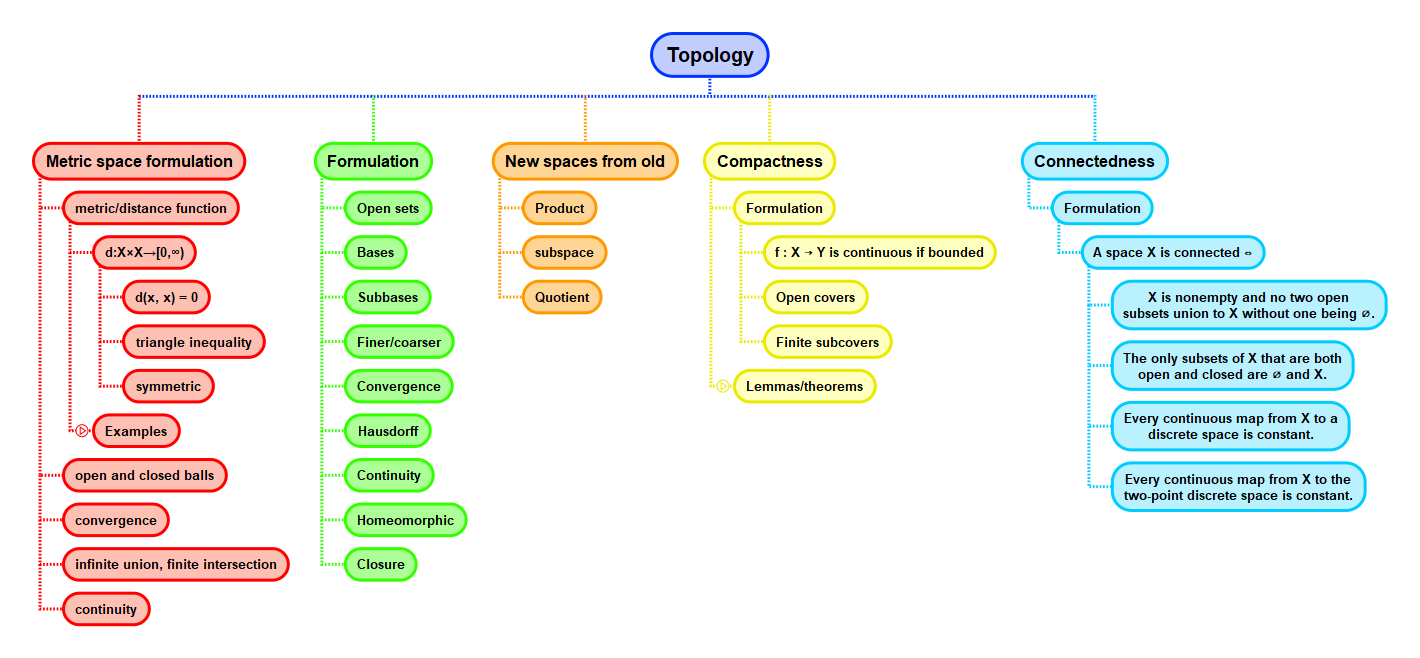Show Question
Math and science::Topology

# Metric space

#### Metric

A metric $$d$$ on a set $$X$$ is a function $$d : X \times X \to [0, \infty)$$ with the following three properties:

What is this called?
$$d(x, y) = 0 \iff x = y, \text{ for all } x, y \in X$$
Triangle inequality
$$d(x, y) + d(y, z) \ge d(x, z), \text{ for all } x, y, z \in X$$
Symmetry
$$d(x, y) = d(y, x), \text{ for all } x, y \in X$$

A metric space is a set together with a metric on it, or more formally, a pair $$(X, d)$$ where $$X$$ is a set and $$d$$ is a metric on $$X$$.

### Example

Some interesting metrics

#### 1. $$d_1, d_2, d_\infty$$ (instances of $$d_p$$) on $$\mathbb{R}^n$$

For all $$x = (x_1, ..., x_n)$$ and $$y = (y_1, ..., y_n)$$ in $$\mathbb{R}^n$$ (or any subset thereof):

• The Euclidean metric, $$d_2$$ on $$\mathbb{R}^n$$ is given by: $$d_2(x, y) = (\sum_{i=1}^{n} (x_i - y_i)^2)^{\frac{1}{2}}$$
• $$d_1$$ is given by: $$d_1(x, y) = \sum_{i=1}^{n}|x_i - y_i|$$
• $$d_\infty$$ by: $$d_\infty(x, y) = max_{1 \le i \le n}|x_i - y_i|$$

All these metrics are particular cases of the $$d_p$$ metric.

#### 2. $$d_1, d_2, d_\infty$$ (instances of $$d_p$$) on $$C[a, b]$$)

All $$d_p$$ metrics have parallels over function spaces. $$C[a, b]$$ is the set of continuous functions $$[a,b] \to \mathbb{R}$$. Three interesting metrics on $$C[a, b]$$ are given by:

$d_1(f, g) = \int_{a}^{b} |f(t) - g(t)|dt$

$d_2(f, g) = \left( \int_{a}^{b} |f(t) - g(t)|dt \right)^{\frac{1}{2}}$

$d_\infty(f, g) = \sup_{a \le t \le b}|f(t) - g(t)|$

#### 3. Hamming metric

With $$A$$ being any set, and $$x, y \in A^n$$, the Hamming metric $$d$$ on $$A$$ is given by

$$d(x, y) = |\{i \in \{1, ..., n\} : x_i \ne y_i \}|$$

In other words, the count of how many coordinates differ.

## Context